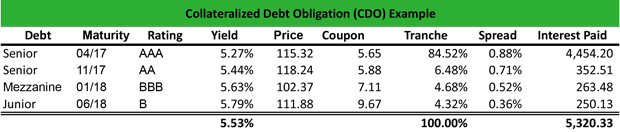# What is a Collateralized Debt Obligation (CDO)?

Definition: Collateralized debt obligations (CDO) are debt instruments, like mortgages, bundled together to create a new type of asset-backed security that can be traded or split. In other words, these are groups of receivables that are insured with an asset.

## What Does Collateralized Debt Obligation Mean?

What is the definition of collateralized debt obligation? CDOs pool together individual fixed-income securities into a structured product and allocate a rate of riskbased on the type of debt. The rule of thumb is that the credit enhancement should be 5 times the expected loss level. Hence, as the rating declines so does the amount of enhancement.

Typically, the CDOs tranches include senior debt (AAA rating), mezzanine debt (AA rating), junior debt (BBB rating), and equity (dividends, yet paid after all the previous holders are paid). Senior tranches receive the highest rating and the lowest coupon rates, whereas junior tranches receive the lowest rating and the higher coupon rates. CDOs use the underlying asset (bond, mortgage or loan) as collateral for protection in case the borrower defaults.

Depending on the type of debt, CDOs are mortgage-backed securities (MBS) when they include mortgage loans or asset-backed securities (ABS) when they trade corporate debt, auto loans or credit card debt.

Let’s look at an example.

## Example

Malcolm invests \$100,000 in a CDO of a total debt 6.15%. The CDO tranches are the following:The senior tranches have AAA and AA ratings and lower coupons than the mezzanine and the junior tranches. Also, the four tranches have different yields with an average yield of 5.53%.

Malcom calculates the spread for each tranche by subtracting the yield from the total debt. Therefore:

1. Senior (AAA) spread = 6.15% – 5.27% = 0.88%
2. Senior (AA) spread = 6.15% – 5.44% = 0.71%
3. Mezzanine spread = 6.15% – 5.63% = 0.52%
4. Junior spread = 6.15% – 5.79% = 0.36%

Then, he calculates the interest that he will pay by multiplying the yield by the size of tranche. Therefore:

1. Senior (AAA) interest = 5.27% x 84.52% x \$100,000 = \$4,454.20
2. Senior (AA) interest = 5.44% x 6.48% x \$100,000 = \$352.51
3. Mezzanine interest = 5.63% x 4.68% x \$100,000 = \$263.48
4. Junior interest = 5.79% x 4.32% x \$100,000 = \$250.13

So, the total interest paid is \$5,320.33.

The interest received is 6.15% + 5.53% x \$100,000 = \$11,682.50

The net profit is \$11,682.50 – \$5,320.33 = \$6,362.17

## Summary Definition

Define Collateralized Debt Obligations: CDO means a structured derivative product that originates their value either by mortgage loans or by corporate debt.